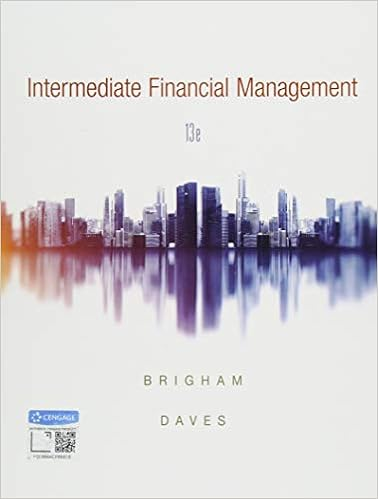Ebit income before tax interest 323 178 501 tax rate

• Notes
• 44
• 93% (208) 194 out of 208 people found this document helpful

This preview shows page 29 - 32 out of 44 pages.

We have textbook solutions for you!
The document you are viewing contains questions related to this textbook.The document you are viewing contains questions related to this textbook.
Chapter 26 / Exercise 26-3
Intermediate Financial Management
Brigham/DavesExpert Verified
EBIT = Income before tax + Interest = 323 + 178 = \$501. Tax rate = 126/323 = .390 or 39.0% Capital expenditures = change in Gross Property and Equipment = 5139 - 4180 = 969 Change in working capital: working capital is Current Assets less operating Current Liabilities—this excludes Short-term debt and Current portion of long-term debt. Hence, the Working Capital balance is 1234 - (77 + 97) = 1060 in 2011, and 931 in 2010. The change in working capital is 1060 - 931 = 129; this increase is a cash outflow. Thus, 2011 Free Cash Flow = 501(1 - .39) + 785 - 969 - 129 = - \$7. [email protected] 11.5% {FCFs, 2011 - 2015} = \$628.24 Terminal value in 2015 = \$6,323.00. [email protected] 11.5% {Terminal value, 5 years} = 3,669.01. Estimated firm value = \$628.24 + 3,669.01 = \$4,297.25
Difficulty: 3 Hard 9-29
We have textbook solutions for you!
The document you are viewing contains questions related to this textbook.The document you are viewing contains questions related to this textbook.
Chapter 26 / Exercise 26-3
Intermediate Financial Management
Brigham/DavesExpert Verified
Chapter 09 - Business Valuation and Corporate Restructuring 14. Assume BSL is worth the book value of its assets at the end of 2015. Macklemore's WACC is 8.0 percent. BSL's WACC is 11.5 percent, and the average of the two companies' WACCs, weighted by sales, is 8.2 percent. What is the maximum acquisition price (in \$ millions) Macklemore should pay to acquire BSL's equity?
Difficulty: 3 Hard 9-30
Chapter 09 - Business Valuation and Corporate Restructuring 15. Estimate BSL's value (in \$ millions) at the end of 2010 assuming that in the years after 2015 the company's free cash flow grows 4 percent per year in perpetuity. Macklemore's WACC is 8.0 percent. BSL's WACC is 11.5 percent, and the average of the two companies' WACCs, weighted by sales, is 8.2 percent. (NOTE to instructor: for this question, you might choose to include the FCF for 2011 of - \$7
•••